Next: ENERGY FLUX IN LAYERED Up: Layers revealed by scattered Previous: Layers revealed by scattered

# REFLECTION AND TRANSMISSION COEFFICIENTS

Consider two halfspaces (the sky above, the earth below). If a wave of unit amplitude is incident onto the boundary, there will be a transmitted wave of amplitude t and a reflected wave of amplitude c as depicted in Figure 1.

 8-1 Figure 1 Waves incident, reflected c, and transmitted t at an interface.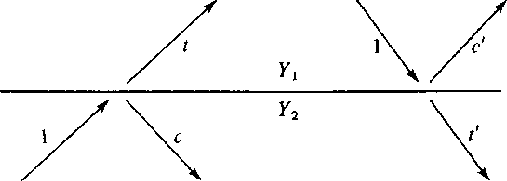A very simple relationship exists between t and c. The wave amplitudes have a physical meaning of something like pressure, material displacement, or tangential electric or magnetic fields; and these physical variables must be the same on either side of the boundary. Thus, we must have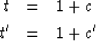(1) (2)

It may be surprising that t may be greater than unity. However, this phenomenon may easily be seen at the ocean, where waves get larger as they approach the shore (until they break). Energy is not determined by wave height alone. Energy is equal to the squared wave amplitude multiplied by a proportionality factor Y depending upon the medium in which the wave is measured. If we denote the factor of the top medium by Y1 and the bottom by Y2, then the statement that the energy before incidence equals the energy after incidence is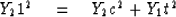(3)
solving for c we get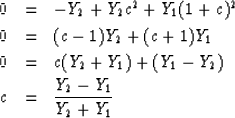(4)

In acoustics the up- and downgoing wave variables may be normalized to either pressure or velocity. When they measure velocity, the scale factor multiplying velocity squared is called the impedance I. When they measure pressure, the scale factor is called the admittance Y.

The wave c' which reflects when energy is incident from the other side is obtained from (4) if Y1 and Y2 are interchanged. Thus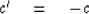(5)

A perfectly reflecting interface is one which does not allow energy through. This comes about not only when t = 0 or c = -1, but also when t = 2 or c = +1. To see this, note that on the left in Figure 1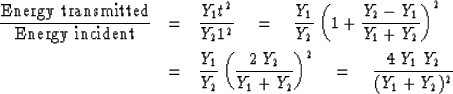(6)

Equation (6) says that 100 percent of the incident energy is transmitted when Y1 = Y2, but the percentage of transmission is very small when Y1 and Y2 are very different.

A word of caution: Occasionally special applications are described by authors who do not define reflection and transmission coefficients in terms of some variable which is continuous at a boundary. This is usually an oversight which unfortunately obscures the relationship of the special application to wave theory in general and this chapter in particular. It is almost never an essential feature of the special application that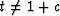but just a result of an unwise choice of variables in the description. For example, material density is an unwise variable in acoustics because it suffers a discontinuity at a material boundary. Pressure or normal velocity are better descriptors of wave strength.

Ordinarily there are two kinds of variables used to describe waves, and both of these can be continuous at a material discontinuity. One is a scalar like pressure, tension, voltage, potential, stress, or temperature. The other is a vector which we use the vertical component. Examples of the latter are velocity, stretch, electric current, dislacement, and heat flow. Occasionally a wave variable will be a tensor. When a boundary condition is the vanishing of one of the motion components, then the boundary is often said to be rigid. When it is the pressure or potential which vanishes, then the boundary is often said to be free. Rigid and free boundaries reflect waves with unit magnitude reflection coefficients.

The purpose of this chapter is to establish fundamental mathematical properties of waves in layers and to avoid specialization to any particular physical type of waves. That will be done in the next chapter. However, so as not to disguise the physical aspect of the mathematics, a precise definition of upgoing wave U and downgoing wave D will now be given in terms of classical acoustics. In acoustics one deals with pressure P and vertical component of parcel velocity W (not to be confused with wave velocity v). One possible definition for U and D (which will be developed in Chapter 9, Section 3) is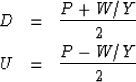(7) (8)
with the inverse relations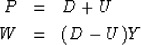(9) (10)

Other definitions with different scale factors and signs are possible. With this definition, the relation t = 1 + c is readily seen to be associated with (9) and continuity of pressure at an interface. The minus signs in (8) and (10) are associated with the direction of the z axis. Reversal of the z axis changes W to -W and switches the roles of U and D.

We notice that a downgoing wave D all by itself with U vanishing provides a moving disturbance of both pressure P and velocity W, and the vanishing of U assures us that the ratio between the two W/P = Y is characteristic admittance Y of the material. The energy, we have said, is proportional to either YP2 or I W2 from which the ratio W/P =Y allows us to deduce that the impedance of a material is the inverse of its admittance I = 1/Y.

 8-2 Figure 2 A waveform R(Z) reflecting at the surface of the sea. Pressure equal to U + D vanishes at the surface. The vertical velocity of the surface is proportional to D - U. Theoretically, waves are observed by measuring W at the surface; however, as a practical matter P is often observed a fraction of a wavelength below the surface.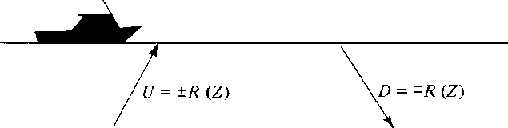For sound waves in the ocean the sea surface is a nearly perfect reflector because of the great contrast between air and water. If this interface is idealized to a perfect reflector, then it is a free surface. Since the pressure vanishes on a free surface, we have that D = -U at the surface so the reflection coefficient is -1. If a wave is to be seen at the surface, it is necessary to measure not pressure but something proportional to velocity. In geophysical exploration practice, pressure sensing hydrophones are used. They must be kept at a suitable distance below the sea surface. The situation can be depicted as in Figure 2. The pressure normally vanishes at the sea surface, but if we wish to initiate an impulsive disturbance, the pressure may momentarily take on some other value, say 1. This is depicted in Figure 3. The total vertical component of velocity of the sea surface due to the source and to the resulting acoustic wave is D - U = 1 + 2R(Z).

 8-3 Figure 3 An initial downgoing disturbance 1 results in a later upgoing reflected wave -R(Z) which reflects back down as R(Z). The pressure at the surface is D + U = 1 + R - R = 1.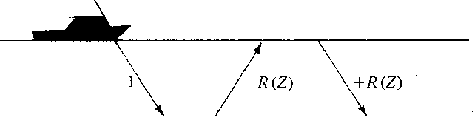## EXERCISES:

1. Compute t in terms of Y1 and Y2.
2. In a certain application continuity is expressed by saying that D-U is the same on either side of the interface. This implies that t = 1 - c. Derive an equation like (4) for the reflection coefficient in terms of the admittance Y.
3. What are reflection and transmission coefficients in terms of the impedance I? (Clear fractions from your result.)
4. From the principle of energy conservation we showed that c' = -c. It may also be deduced from time reversal. To do this, copy Figure 1 with arrows reversed. Scale and linearly superpose various figures in an attempt to create a situation where a figure like the right-hand side of Figure 1 has -c' for the reflected wave. (HINT: Draw arrows at normal incidence.)Next: ENERGY FLUX IN LAYERED Up: Layers revealed by scattered Previous: Layers revealed by scattered
Stanford Exploration Project
10/30/1997